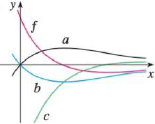Chapter 4.9, Problem 52E### Single Variable Calculus: Early Tr...

8th Edition
James Stewart
ISBN: 9781305270343

#### Solutions

Chapter
Section### Single Variable Calculus: Early Tr...

8th Edition
James Stewart
ISBN: 9781305270343
Textbook Problem

# The graph of a function f is shown. Which graph is an antiderivative of f and why?To determine

To identify: The graph of antiderivative for the function f in the given graph.

Explanation

Given Data:

Refer to the Figure in the textbook for the given graph.

The conditions for a particular graph x to be an antiderivative for the function f are written as follows.

• The graph x increases when the function f is positive and
• The graph x decreases when the function f is negative.

Observation:

From the given graph in the textbook, when the function f is positive (for small values of x ), the graph b is decreasing and the graph a is increasing

### Still sussing out bartleby?

Check out a sample textbook solution.

See a sample solution

#### The Solution to Your Study Problems

Bartleby provides explanations to thousands of textbook problems written by our experts, many with advanced degrees!

Get Started

#### In Exercises 1-6, simplify the expression. 2. 2a23ab9b22ab2+3b3

Applied Calculus for the Managerial, Life, and Social Sciences: A Brief Approach

#### In problems 63-73, factor each expression completely. 63.

Mathematical Applications for the Management, Life, and Social Sciences

#### Find the derivative of the function. y=x+x+x

Single Variable Calculus: Early Transcendentals

#### Simplify each expression. 3

Trigonometry (MindTap Course List)

#### In Exercises 110, find the graphical solution to each inequality. y3

Finite Mathematics for the Managerial, Life, and Social Sciences

#### For y = x sin x, y = _____. a) x cos x b) x cos x + 1 c) cos x d) x cos x + sin x

Study Guide for Stewart's Single Variable Calculus: Early Transcendentals, 8th